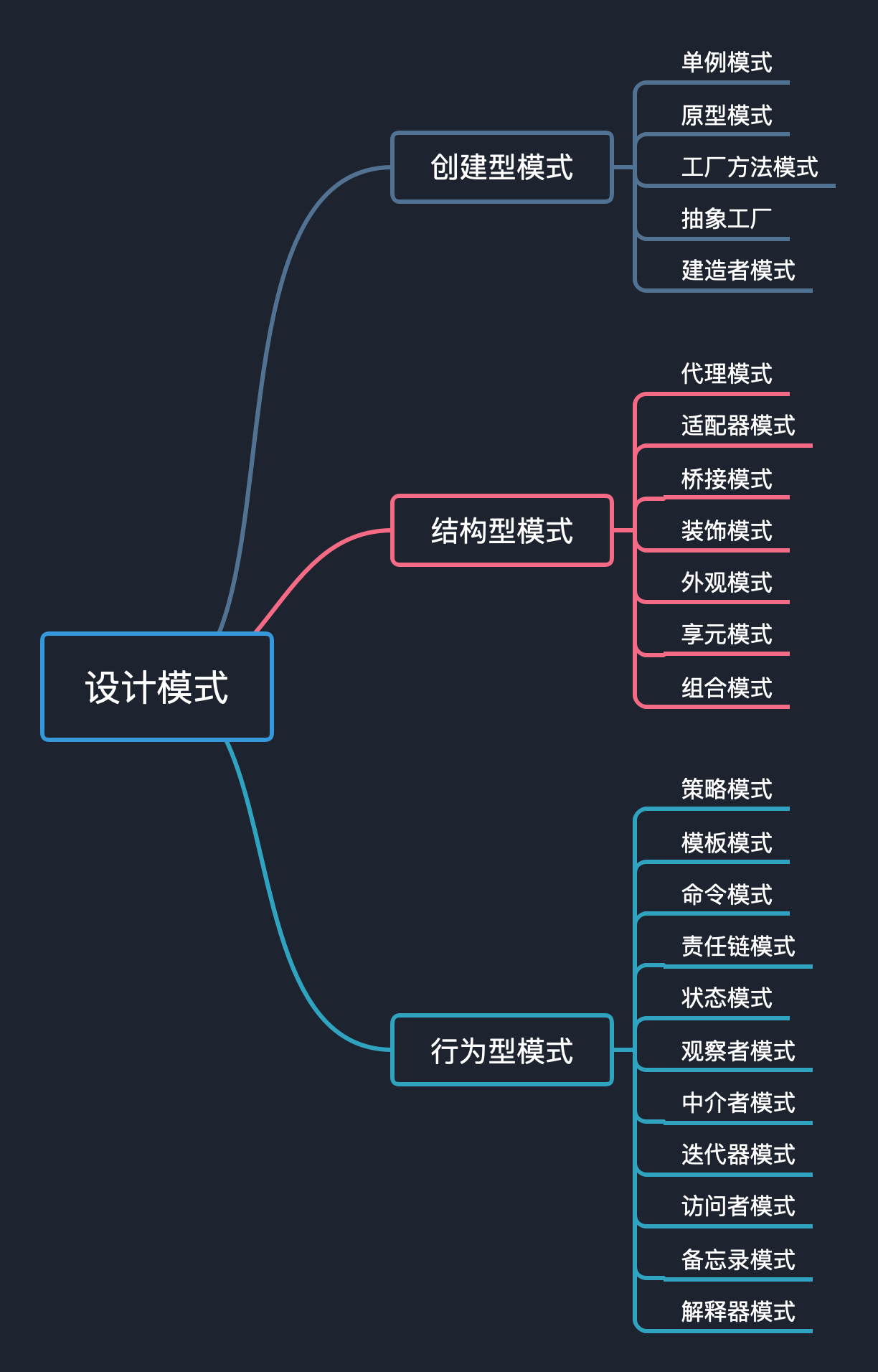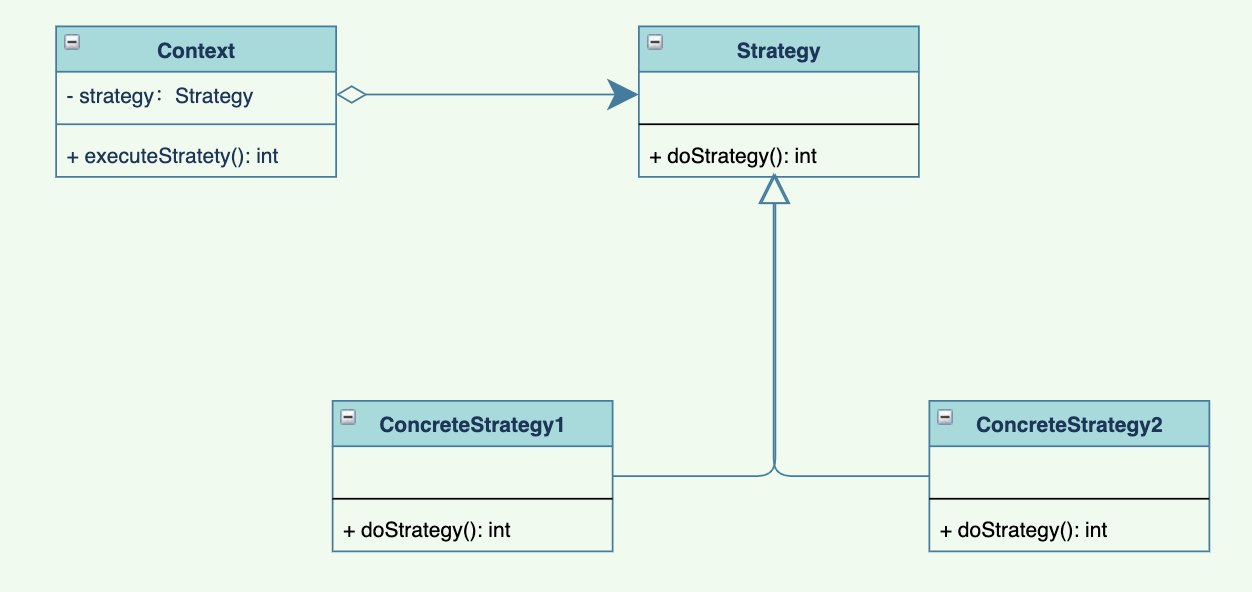• 累计撰写 145 篇文章
• 累计创建 37 个标签
• 累计收到 18 条评论

### 目 录CONTENT# 设计模式【12】-- 搞定最近大火的策略模式2022-01-23 / 0 评论 / 0 点赞 / 585 阅读 / 4,818 字## 策略模式到底是什么？

• 创建型模式：如何创建一个对象
• 结构型模式：对象内部的构造是如何构造的
• 行为型模式：对象是如何运行（可以做什么）

• 从北京到上海，可以坐飞机，也可以坐动车，也可以坐绿皮，甚至可以骑自行车，这些不同的方式，就是策略。

• 一件商品，可以直接打 5 折，也可以加 100 再打 6 折，也可以打 5.5 折之后返回现金券，这些也是策略。

• 带了 200 去超市买东西，可以买零食，也可以买生活用品，也可以啥也不买，这些也是策略。

## 策略模式的角色

• 抽象的策略类（Strategy）：将策略的行为抽象出公共接口
• 具体的策略类（ConcreteStrategy）:以Strategy接口实现某些具体的算法，具体的策略可能多个，也可以相互替换
• 上下文（Context）: 一般是封装了策略，以及策略的使用方法，对上层调用屏蔽了细节，直接调用即可。## 策略模式的demo

public interface Strategy {

int doStrategy();
}



public class ConcreteStrategy1 implements Strategy{
@Override
public int doStrategy() {
System.out.println("执行策略1...");
return 1;
}
}


public class ConcreteStrategy2 implements Strategy{
@Override
public int doStrategy() {
System.out.println("执行策略2...");
return 2;
}
}



public class Context {
private Strategy strategy;

public Context(Strategy strategy) {
this.strategy = strategy;
}

public void executeStrategy(){
strategy.doStrategy();
}
}


public class Test {
public static void main(String[] args) {
Context context1 = new Context(new ConcreteStrategy1());
context1.executeStrategy();

context1 = new Context(new ConcreteStrategy2());
context1.executeStrategy();
}
}



执行策略1



## 分红包策略实战

public class RedPackage {
public int remainSize;

public int remainMoney;

public RedPackage(int remainSize, int remainMoney) {
this.remainSize = remainSize;
this.remainMoney = remainMoney;
}
}


import java.util.List;

public interface AllocateStrategy {

List<Integer> allocate(RedPackage redPackage);

}


import java.util.ArrayList;
import java.util.List;

public class AverageAllocateStrategy implements AllocateStrategy {
@Override
public List<Integer> allocate(RedPackage redPackage) {
List<Integer> results = new ArrayList<>();
Integer sum = redPackage.remainMoney;
Integer average = sum / redPackage.remainSize;
for (int i = 0; i < redPackage.remainSize; i++) {
sum = sum - average;
}
if (sum != 0) {
results.set(0, results.get(0) + sum);
}
return results;
}
}




import java.util.ArrayList;
import java.util.List;
import java.util.Random;

public class RandomAllocateStrategy implements AllocateStrategy {
@Override
public List<Integer> allocate(RedPackage redPackage) {
return ranRedPackage(redPackage.remainSize, redPackage.remainMoney);
}

public List<Integer> ranRedPackage(Integer count, Integer money) {
List<Integer> result = new ArrayList<>();
for (int i = 0; i < count; i++) {
}
for (int i = 1; i <= money; i++) {
int n = new Random().nextInt(count);
result.set(n, result.get(n) + 1);
}
return result;
}

}



import java.util.List;

public class Context {
private AllocateStrategy strategy;

public Context(AllocateStrategy strategy) {
this.strategy = strategy;
}

public List<Integer> executeStrategy(RedPackage redPackage){
return strategy.allocate(redPackage);
}
}



import java.util.List;

public class Test {
public static void main(String[] args) {
RedPackage redPackage = new RedPackage(10,102);
Context context = new Context(new RandomAllocateStrategy());
List<Integer> list =context.executeStrategy(redPackage);
list.forEach(l-> System.out.print(l+" "));

System.out.println("");
context = new Context(new AverageAllocateStrategy());
list =context.executeStrategy(redPackage);
list.forEach(l-> System.out.print(l+" "));
}
}



9 10 16 8 14 8 7 15 9 6
12 10 10 10 10 10 10 10 10 10


## 优缺点

• 消除if-else语句，不同策略相互隔离，方便维护
• 切换自由
• 拓展性好，实现接口即可

• 策略一旦数量过多，维护也会相对比较难，复用代码比较难
• 所有的策略类都对外暴露了，虽然一般通过Context上下文调用

【作者简介】

0